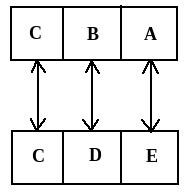# The six faces of a dice have been marked with alphabets A, B, C, D, E and F respectively. This dice is rolled down three times. The three positions are shown as:Find the alphabet opposite A.   Option 1)   A   Option 2)   D   Option 3)   E   Option 4)   F   Option 5)   C

In the above three diagrams, These are not a numeric dice. So, here in this case,

To find out the opposite faces we will wright all the elements in the clockwise direction starting with the common element in any two figure.

So taking figure (i) & figure (ii) for the above experiment,Letter Opposite to A --------> E

Exams
Articles
Questions